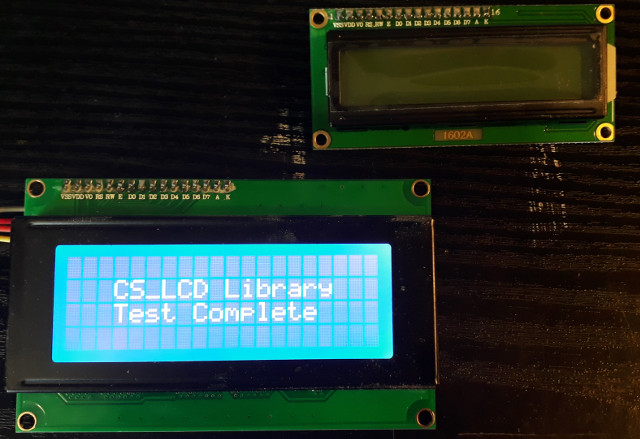"... and no one shall work for money, and no one shall work for fame; But each for the joy of the working, and each, in his separate star, shall draw the thing as he sees it, for the god of things as they are"

-Kipling

# CS_LCD:A C# Library for LCD Displays (HD44780 Compatible) via I2C

### Summary

The purpose of this project is to provide a C# based library which can control a LCD screen via I2C. This library is compatible(ish) in functionality with the popular Arduino Liquid Crystal Library - the function calls operate in the same way but there have been some name changes. A test suite for C# on the Meadow F7 board and the Beaglebone Black running the BBBCSIO I/O library is also provided. The architecture of the projects is such that it would be simple to adapt the code to other platforms and to data transmission mechanisms other than I2C.

### Usage

To use the library just reference the CS_LCD.dll in your project or, since the full source code is provided, include the CS_LCD project in your C# solution.

### The CS_LCD Library Calls

The following calls are publicly available in the CS_LCD library.

```        /// +=+=+=+=+=+=+=+=+=+=+=+=+=+=+=+=+=+=+=+=+=+=+=+=+=+=+=+=+=+=+=+=+=+=+=+=
/// <summary>
/// Constructor
/// </summary>
/// <param name="numRowsIn">the number of rows on the display</param>
/// <param name="numColsIn">the number of cols on the display</param>
protected CS_LCDBase(int numRowsIn, int numColsIn)

/// +=+=+=+=+=+=+=+=+=+=+=+=+=+=+=+=+=+=+=+=+=+=+=+=+=+=+=+=+=+=+=+=+=+=+=+=
/// <summary>
/// Initializes and resets the LCD
/// </summary>
public void InitLCD()

/// +=+=+=+=+=+=+=+=+=+=+=+=+=+=+=+=+=+=+=+=+=+=+=+=+=+=+=+=+=+=+=+=+=+=+=+=
/// <summary>
/// Clears the LCD display and moves the cursor to the top left corner.
/// </summary>
public void Clear()

/// +=+=+=+=+=+=+=+=+=+=+=+=+=+=+=+=+=+=+=+=+=+=+=+=+=+=+=+=+=+=+=+=+=+=+=+=
/// <summary>
/// Causes the cursor to be made visible.
/// </summary>
public void ShowCursor()

/// +=+=+=+=+=+=+=+=+=+=+=+=+=+=+=+=+=+=+=+=+=+=+=+=+=+=+=+=+=+=+=+=+=+=+=+=
/// <summary>
/// Causes the cursor to be hidden.
/// </summary>
public void HideCursor()

/// +=+=+=+=+=+=+=+=+=+=+=+=+=+=+=+=+=+=+=+=+=+=+=+=+=+=+=+=+=+=+=+=+=+=+=+=
/// <summary>
/// Turns on the cursor, and makes it blink.
/// </summary>

/// +=+=+=+=+=+=+=+=+=+=+=+=+=+=+=+=+=+=+=+=+=+=+=+=+=+=+=+=+=+=+=+=+=+=+=+=
/// <summary>
/// Turns on the cursor, and makes it not blink (i.e. be solid)
/// </summary>

/// +=+=+=+=+=+=+=+=+=+=+=+=+=+=+=+=+=+=+=+=+=+=+=+=+=+=+=+=+=+=+=+=+=+=+=+=
/// <summary>
/// Turns on (i.e. unblanks) the LCD.
/// </summary>
public void DisplayOn()

/// +=+=+=+=+=+=+=+=+=+=+=+=+=+=+=+=+=+=+=+=+=+=+=+=+=+=+=+=+=+=+=+=+=+=+=+=
/// <summary>
/// Turns off (i.e. blanks) the LCD.
/// </summary>
public void DisplayOff()

/// +=+=+=+=+=+=+=+=+=+=+=+=+=+=+=+=+=+=+=+=+=+=+=+=+=+=+=+=+=+=+=+=+=+=+=+=
/// <summary>
/// Turns the backlight on.
/// </summary>
public void BacklightOn()

/// +=+=+=+=+=+=+=+=+=+=+=+=+=+=+=+=+=+=+=+=+=+=+=+=+=+=+=+=+=+=+=+=+=+=+=+=
/// <summary>
/// Turns the backlight off.
/// </summary>
public void BacklightOff()

/// +=+=+=+=+=+=+=+=+=+=+=+=+=+=+=+=+=+=+=+=+=+=+=+=+=+=+=+=+=+=+=+=+=+=+=+=
/// <summary>
/// Writes the indicated character to the LCD at the current cursor
//     position, and advances the cursor by one position
/// </summary>
/// <param name="inChar">the character to write</param>
public void WriteChar(char inChar)

/// +=+=+=+=+=+=+=+=+=+=+=+=+=+=+=+=+=+=+=+=+=+=+=+=+=+=+=+=+=+=+=+=+=+=+=+=
/// <summary>
/// Write the indicated string to the LCD at the current cursor
/// position and advances the cursor position appropriately.
/// </summary>
/// <param name="inStr">the string to write</param>
public void WriteString(string inStr)

/// +=+=+=+=+=+=+=+=+=+=+=+=+=+=+=+=+=+=+=+=+=+=+=+=+=+=+=+=+=+=+=+=+=+=+=+=
/// <summary>
/// Write a character to one of the 8 CGRAM locations, available
///    as 0x00 through 0x07
///
/// Note: Once the custom char is set you use it by writing 0x00 to 0x07
///       as the character. IE instead of sending 'A' (or 0x41) to draw
///       an 'A' on the screen you would send 0x00 to display the custom
///       character placed in that location by this call.
/// </summary>
/// <param name="location">the location, this can only be 0x00 to 0x07</param>
/// <param name="byteArr">eight bytes which describe the pixels in the character
/// only the low 5 bits are significant</param>
public void CustomChar(byte location, byte[] byteArr)

/// +=+=+=+=+=+=+=+=+=+=+=+=+=+=+=+=+=+=+=+=+=+=+=+=+=+=+=+=+=+=+=+=+=+=+=+=
/// <summary>
/// Set the cursor position. Note the Arduino Liquid_Crystal library uses
/// a reverse ordering (col, row) of the parameters in its equivalent function
///
/// Note: if the position is set out of bounds the cursor will be set to the
/// closest boundary. The cursor position is zero based (i.e. col == 0 indicates
/// the first column)
/// </summary>
public void MoveTo(int row, int col)

/// +=+=+=+=+=+=+=+=+=+=+=+=+=+=+=+=+=+=+=+=+=+=+=+=+=+=+=+=+=+=+=+=+=+=+=+=
/// <summary>
/// Gets/Sets the currentCursorRow on the LCD.  Cursor is always
/// zero based. High bounds are not checked so the calling code can implement
/// wrapping as desired.
///
/// </summary>
public int CurrentCursorRow

/// +=+=+=+=+=+=+=+=+=+=+=+=+=+=+=+=+=+=+=+=+=+=+=+=+=+=+=+=+=+=+=+=+=+=+=+=
/// <summary>
/// Gets/Sets the currentCursorCol on the LCD.  Cursor is always
/// zero based. High bounds are not checked so the calling code can implement
/// wrapping as desired.
///
/// </summary>
public int CurrentCursorCol

/// +=+=+=+=+=+=+=+=+=+=+=+=+=+=+=+=+=+=+=+=+=+=+=+=+=+=+=+=+=+=+=+=+=+=+=+=
/// <summary>
/// Gets/Sets the number of rows on the LCD. Zero based.
///
/// </summary>
public int NumRows

/// +=+=+=+=+=+=+=+=+=+=+=+=+=+=+=+=+=+=+=+=+=+=+=+=+=+=+=+=+=+=+=+=+=+=+=+=
/// <summary>
/// Gets/Sets the number of cols on the LCD. Zero based.
///
/// </summary>
public int NumCols
```

### The Sample Code

The CS_LCD Library and Sample Applications are open source and released under the MIT License. You can download, clone or fork the CS_LCD Sample Projects at the following address:

### Screenshots

The example screenshot below is of a 4 row by x 20 column (i.e. a 2004) LCD having completed the test suite on the Beaglebone Black.### Acknowledgements

The CS_LCD library source is based on, and largely ported from, the excellent python_lcd code of Dave Hylands https://github.com/dhylands/python_lcd